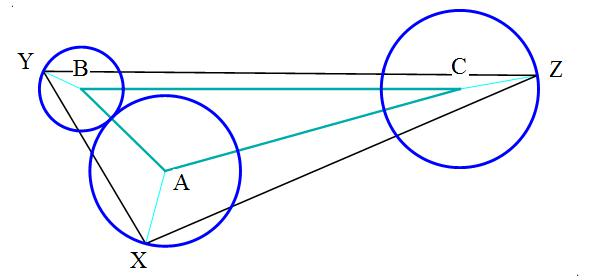# A Flock Of Herons

Geometry Level 5

Triangles $\Delta ABC$ and $\Delta XYZ$ are Heronian triangles, which are triangles that have integer sides with integer areas.Points $A,B,C$ are centers of circles, the radii of which are

Radius of circle $A=1092=2^2\cdot 3\cdot 7\cdot 13$
Radius of circle $B=608=2^5\cdot 19$
Radius of circle $C=1140=2^2\cdot 3\cdot 5\cdot 19$

The sides of triangle $\Delta ABC$ are

$AB=1700=2^2\cdot 5^2\cdot 17$
$BC=5491=17^2\cdot 19$
$CA=4437=3^2\cdot 17\cdot 29$

Triangle $\Delta XYZ$ has the maximum area of any triangle (Heronian or not) that has one vertex on the circumference of each of the circles $A,B,C$

Let $\dfrac { \Delta XYZ }{ \Delta ABC } =\dfrac { p }{ q }$

be the ratio of the areas of triangles $\Delta XYZ$ and $\displaystyle \Delta ABC$

where $p,q$ are $4$ digit co-prime integers. The difference $p-q$ is a $4$ digit prime number.

Find that prime number.

For your convenience, area of triangle $\displaystyle \Delta ABC$ works out to

$3261654=2\cdot 3^3\cdot 11\cdot 17^2\cdot 19$

Note: Triangle $\Delta XYZ$ as drawn in graphic does not have the maximum area

Also, as you solve this, you'll come across more Herons. A helpful hint, I hope.

×

Problem Loading...

Note Loading...

Set Loading...